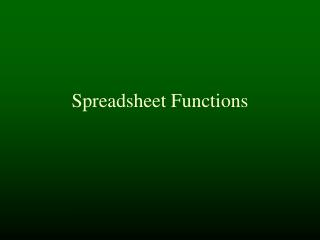Download Presentation# Spreadsheet Functions - PowerPoint PPT PresentationDownload Presentation- - - - - - - - - - - - - - - - - - - - - - - - - - - E N D - - - - - - - - - - - - - - - - - - - - - - - - - - -
##### Presentation Transcript

2. Functions • Simple functions • Array functions • IF - logical functions

3. Function notation • A function is a piece of computation that takes 1 or more arguments (data) and returns a value • Function( arg1, arg2, arg3 ) • Sine(90) • Max(100,200,120,-7) • Sqrt( min(7+4, 9) )

4. Using functions

5. Using functions

6. Finding functions

7. Finding functions

8. Finding functions

9. Finding functions

10. Finding functions

11. Finding functions

12. Functions • Simple functions • Array functions • IF - logical functions

13. Sum

14. Sum program Sum=0; for (each row R) { for (each column C in row R) { Sum=Sum+Sheet[R, C]; } }

15. Sum program Sum=0; for (each row R) { for (each column C in row R) { Sum=Sum+Sheet[R, C]; } }

16. Sum program Sum=0; for (each row R) { for (each column C in row R) { Sum=Sum+Sheet[R, C]; } }

17. Sum program Sum=0; for (each row R) { for (each column C in row R) { Sum=Sum+Sheet[R, C]; } }

18. Sum program Sum=0; for (each row R) { for (each column C in row R) { Sum=Sum+Sheet[R, C]; } }

19. Sum program Sum=0; for (each row R) { for (each column C in row R) { Sum=Sum+Sheet[R, C]; } }

20. Sum program Sum=0; for (each row R) { for (each column C in row R) { Sum=Sum+Sheet[R, C]; } }

21. Sum program Sum=0; for (each row R) { for (each column C in row R) { Sum=Sum+Sheet[R, C]; } }

22. Sum program Sum=0; for (each row R) { for (each column C in row R) { Sum=Sum+Sheet[R, C]; } }

23. Sum program Sum=0; for (each row R) { for (each column C in row R) { Sum=Sum+Sheet[R, C]; } }

24. Sum program Sum=0; for (each row R) { for (each column C in row R) { Sum=Sum+Sheet[R, C]; } }

25. Sum program Sum=0; for (each row R) { for (each column C in row R) { Sum=Sum+Sheet[R, C]; } }

26. Sum program Sum=0; for (each row R) { for (each column C in row R) { Sum=Sum+Sheet[R, C]; } }

27. Sum program Sum=0; for (each row R) { for (each column C in row R) { Sum=Sum+Sheet[R, C]; } }

28. Sum program Sum=0; for (each row R) { for (each column C in row R) { Sum=Sum+Sheet[R, C]; } }

29. Sum program Sum=0; for (each row R) { for (each column C in row R) { Sum=Sum+Sheet[R, C]; } }

30. Count

31. Count program Count=0; for (each row R) { for (each column C in row R) { Count=Count+1; } }

32. Average

33. Average Program

34. Average program Sum=0; Count = 0; for (each row R) { for (each column C in row R) { Sum=Sum+Sheet[R,C]; Count = Count+1; } } Average = Sum/Count;

35. Other array functions • Min • Max • Stdev - standard deviation • Median • Product - multiply them all together

36. Functions • Simple functions • Array functions • IF - logical functions

37. If • If ( test, trueVal, falseVal)

38. AND

39. IF with AND

40. Review • Functions - name with arguments • Finding functions • Array functions • Sum(B6:D32) • Average(F14:R75) • IF - AND - OR